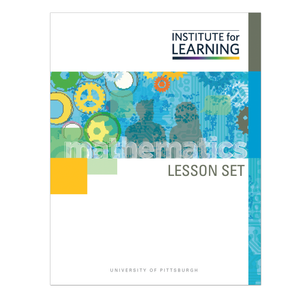# Comparing Fractions with Common Denominators or Common Numerators (Grade 3)

Regular price \$6.25 \$0.00 Unit price per

This lesson set is designed to provide students opportunities to compare and reason about the comparison of fractions with either common denominators or common numerators. Students will:

• solve real-world situations that require a comparison of fractional amounts;
• reason about the meaning of the numerator and denominator to compare fractions;
• work with fractional amounts between 0 and 1 and fractional amounts greater than or equal to 1; and
• create and use regional and number line models to represent and compare fractions.

Standards: 3.NF.A.1, 3.NF.A.2, 3.NF.A.2.A, 3.NF.A.2.B, 3.NF.A.3, 3.NF.A.3.A, 3.NF.A.3.B, 3.NF.A.3.C, and 3.NF.A.3.D